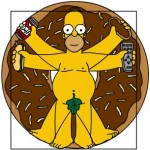What does Pi Sound Like?According to Wikipedia, Pi is is a mathematical constant whose value is the ratio of any Euclidean plane circle’s circumference to its diameter; this is the same value as the ratio of a circle’s area to the square of its radius. It is approximately equal to 3.14159265 in the usual decimal notation. Many formulae from mathematics, science, and engineering involve Pi, which makes it one of the most important mathematical constants.

Michael John Blake a jazz saxophonist a member of the the Lounge Lizards from 1990, assigned a music cord to a number and then played the notes to the value of Pi…this is what it sounds like:

You can buy the music on iTunes – “What Pi Sounds Like

MadMike posted at 2011-3-16 Category: Interesting Finds